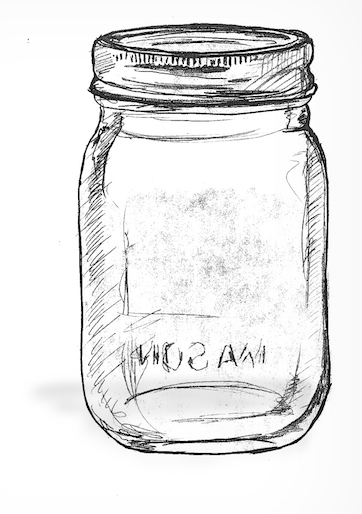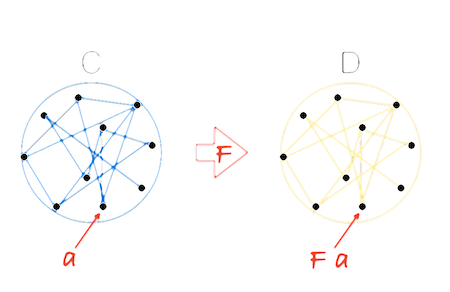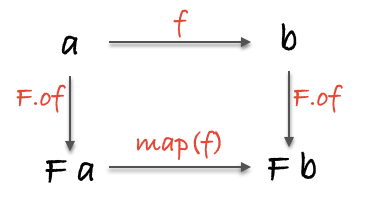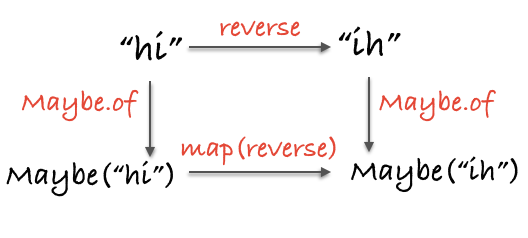# 特百惠

（译者注：特百惠是美国家居用品品牌，代表产品是塑料容器。）

## 强大的容器``````var Container = function(x) {
this.__value = x;
}

Container.of = function(x) { return new Container(x); };
``````

``````Container.of(3)
//=> Container(3)

Container.of("hotdogs")
//=> Container("hotdogs")

Container.of(Container.of({name: "yoda"}))
//=> Container(Container({name: "yoda" }))
``````

• `Container` 是个只有一个属性的对象。尽管容器可以有不止一个的属性，但大多数容器还是只有一个。我们很随意地把 `Container` 的这个属性命名为 `__value`
• `__value` 不能是某个特定的类型，不然 `Container` 就对不起它这个名字了。
• 数据一旦存放到 `Container`，就会一直待在那儿。我们可以`.__value` 获取到数据，但这样做有悖初衷。

## 第一个 functor

``````// (a -> b) -> Container a -> Container b
Container.prototype.map = function(f){
return Container.of(f(this.__value))
}
``````

``````Container.of(2).map(function(two){ return two + 2 })
//=> Container(4)

Container.of("flamethrowers").map(function(s){ return s.toUpperCase() })
//=> Container("FLAMETHROWERS")

Container.of("bombs").map(concat(' away')).map(_.prop('length'))
//=> Container(10)
``````

functor 是实现了 `map` 函数并遵守一些特定规则的容器类型。

## 薛定谔的 Maybe``````var Maybe = function(x) {
this.__value = x;
}

Maybe.of = function(x) {
return new Maybe(x);
}

Maybe.prototype.isNothing = function() {
return (this.__value === null || this.__value === undefined);
}

Maybe.prototype.map = function(f) {
return this.isNothing() ? Maybe.of(null) : Maybe.of(f(this.__value));
}
``````

`Maybe` 看起来跟 `Container` 非常类似，但是有一点不同：`Maybe` 会先检查自己的值是否为空，然后才调用传进来的函数。这样我们在使用 `map` 的时候就能避免恼人的空值了（注意这个实现出于教学目的做了简化）。

``````Maybe.of("Malkovich Malkovich").map(match(/a/ig));
//=> Maybe(['a', 'a'])

Maybe.of(null).map(match(/a/ig));
//=> Maybe(null)

//=> Maybe(null)

//=> Maybe(24)
``````

``````//  map :: Functor f => (a -> b) -> f a -> f b
var map = curry(function(f, any_functor_at_all) {
return any_functor_at_all.map(f);
});
``````

## 用例

``````//  safeHead :: [a] -> Maybe(a)
return Maybe.of(xs);
};

// Maybe(null)

``````

`safeHead` 与一般的 `_.head` 类似，但是增加了类型安全保证。引入 `Maybe` 会发生一件非常有意思的事情，那就是我们被迫要与狡猾的 `null` 打交道了。`safeHead` 函数能够诚实地预告它可能的失败——失败真没什么可耻的——然后返回一个 `Maybe` 来通知我们相关信息。实际上不仅仅是通知，因为毕竟我们想要的值深藏在 `Maybe` 对象中，而且只能通过 `map` 来操作它。本质上，这是一种由 `safeHead` 强制执行的空值检查。有了这种检查，我们才能在夜里安然入睡，因为我们知道最不受人待见的 `null` 不会突然出现。类似这样的 API 能够把一个像纸糊起来的、脆弱的应用升级为实实在在的、健壮的应用，这样的 API 保证了更加安全的软件。

``````//  withdraw :: Number -> Account -> Maybe(Account)
var withdraw = curry(function(amount, account) {
return account.balance >= amount ?
Maybe.of({balance: account.balance - amount}) :
Maybe.of(null);
});

//  finishTransaction :: Account -> String
var finishTransaction = compose(remainingBalance, updateLedger); // <- 假定这两个函数已经在别处定义好了

//  getTwenty :: Account -> Maybe(String)
var getTwenty = compose(map(finishTransaction), withdraw(20));

getTwenty({ balance: 200.00});

getTwenty({ balance: 10.00});
// Maybe(null)
``````

## 释放容器里的值

``````//  maybe :: b -> (a -> b) -> Maybe a -> b
var maybe = curry(function(x, f, m) {
return m.isNothing() ? x : f(m.__value);
});

//  getTwenty :: Account -> String
var getTwenty = compose(
maybe("You're broke!", finishTransaction), withdraw(20)
);

getTwenty({ balance: 200.00});

getTwenty({ balance: 10.00});
// "You're broke!"
``````

## “纯”错误处理``````var Left = function(x) {
this.__value = x;
}

Left.of = function(x) {
return new Left(x);
}

Left.prototype.map = function(f) {
return this;
}

var Right = function(x) {
this.__value = x;
}

Right.of = function(x) {
return new Right(x);
}

Right.prototype.map = function(f) {
return Right.of(f(this.__value));
}
``````

`Left``Right` 是我们称之为 `Either` 的抽象类型的两个子类。我略去了创建 `Either` 父类的繁文缛节，因为我们不会用到它的，但你了解一下也没坏处。注意看，这里除了有两个类型，没别的新鲜东西。来看看它们是怎么运行的：

``````Right.of("rain").map(function(str){ return "b"+str; });
// Right("brain")

Left.of("rain").map(function(str){ return "b"+str; });
// Left("rain")

Right.of({host: 'localhost', port: 80}).map(_.prop('host'));
// Right('localhost')

Left.of("rolls eyes...").map(_.prop("host"));
// Left('rolls eyes...')
``````

`Left` 就像是青春期少年那样无视我们要 `map` 它的请求。`Right` 的作用就像是一个 `Container`（也就是 Identity）。这里强大的地方在于，`Left` 有能力在它内部嵌入一个错误消息。

``````var moment = require('moment');

//  getAge :: Date -> User -> Either(String, Number)
var getAge = curry(function(now, user) {
var birthdate = moment(user.birthdate, 'YYYY-MM-DD');
if(!birthdate.isValid()) return Left.of("Birth date could not be parsed");
return Right.of(now.diff(birthdate, 'years'));
});

getAge(moment(), {birthdate: '2005-12-12'});
// Right(9)

getAge(moment(), {birthdate: 'balloons!'});
// Left("Birth date could not be parsed")
``````

``````//  fortune :: Number -> String
var fortune  = compose(concat("If you survive, you will be "), add(1));

//  zoltar :: User -> Either(String, _)
var zoltar = compose(map(console.log), map(fortune), getAge(moment()));

zoltar({birthdate: '2005-12-12'});
// "If you survive, you will be 10"
// Right(undefined)

zoltar({birthdate: 'balloons!'});
// Left("Birth date could not be parsed")
``````

`Either` 并不仅仅只对合法性检查这种一般性的错误作用非凡，对一些更严重的、能够中断程序执行的错误比如文件丢失或者 socket 连接断开等，`Either` 同样效果显著。你可以试试把前面例子中的 `Maybe` 替换为 `Either`，看怎么得到更好的反馈。

``````//  either :: (a -> c) -> (b -> c) -> Either a b -> c
var either = curry(function(f, g, e) {
switch(e.constructor) {
case Left: return f(e.__value);
case Right: return g(e.__value);
}
});

//  zoltar :: User -> _
var zoltar = compose(console.log, either(id, fortune), getAge(moment()));

zoltar({birthdate: '2005-12-12'});
// "If you survive, you will be 10"
// undefined

zoltar({birthdate: 'balloons!'});
// "Birth date could not be parsed"
// undefined
``````

## 王老先生有作用...``````//  getFromStorage :: String -> (_ -> String)
var getFromStorage = function(key) {
return function() {
return localStorage[key];
}
}
``````

``````var IO = function(f) {
this.__value = f;
}

IO.of = function(x) {
return new IO(function() {
return x;
});
}

IO.prototype.map = function(f) {
return new IO(_.compose(f, this.__value));
}
``````

`IO` 跟之前的 functor 不同的地方在于，它的 `__value` 总是一个函数。不过我们不把它当作一个函数——实现的细节我们最好先不管。这里发生的事情跟我们在 `getFromStorage` 那里看到的一模一样：`IO` 把非纯执行动作（impure action）捕获到包裹函数里，目的是延迟执行这个非纯动作。就这一点而言，我们认为 `IO` 包含的是被包裹的执行动作的返回值，而不是包裹函数本身。这在 `of` 函数里很明显：`IO(function(){ return x })` 仅仅是为了延迟执行，其实我们得到的是 `IO(x)`

``````//  io_window_ :: IO Window
var io_window = new IO(function(){ return window; });

io_window.map(function(win){ return win.innerWidth });
// IO(1430)

io_window.map(_.prop('location')).map(_.prop('href')).map(split('/'));
// IO(["http:", "", "localhost:8000", "blog", "posts"])

//  \$ :: String -> IO [DOM]
var \$ = function(selector) {
return new IO(function(){ return document.querySelectorAll(selector); });
}

// IO('I am some inner html')
``````

``````
////// 纯代码库: lib/params.js ///////

//  url :: IO String
var url = new IO(function() { return window.location.href; });

//  toPairs =  String -> [[String]]
var toPairs = compose(map(split('=')), split('&'));

//  params :: String -> [[String]]
var params = compose(toPairs, last, split('?'));

//  findParam :: String -> IO Maybe [String]
var findParam = function(key) {
return map(compose(Maybe.of, filter(compose(eq(key), head)), params), url);
};

////// 非纯调用代码: main.js ///////

// 调用 __value() 来运行它！
findParam("searchTerm").__value();
// Maybe(['searchTerm', 'wafflehouse'])
``````

lib/params.js 把 `url` 包裹在一个 `IO` 里，然后把这头野兽传给了调用者；一双手保持的非常干净。你可能也注意到了，我们把容器也“压栈”了，要知道创建一个 `IO(Maybe([x]))` 没有任何不合理的地方。我们这个“栈”有三层 functor（`Array` 是最有资格成为 mappable 的容器类型），令人印象深刻。

``````var IO = function(f) {
this.unsafePerformIO = f;
}

IO.prototype.map = function(f) {
return new IO(_.compose(f, this.unsafePerformIO));
}
``````

`IO` 会成为一个忠诚的伴侣，帮助我们驯化那些狂野的非纯操作。下一节我们将学习一种跟 `IO` 在精神上相似，但是用法上又千差万别的类型。

## 异步任务

``````// Node readfile example:
//=======================

var fs = require('fs');

err ? reject(err) : result(data);
});
});
};

// Task("One morning, as Gregor Samsa was waking up from anxious dreams, he discovered that
// in bed he had been changed into a monstrous verminous bug.")

// jQuery getJSON example:
//========================

//  getJSON :: String -> {} -> Task(Error, JSON)
var getJSON = curry(function(url, params) {
\$.getJSON(url, params, result).fail(reject);
});
});

getJSON('/video', {id: 10}).map(_.prop('title'));

// 传入普通的实际值也没问题
Task.of(3).map(function(three){ return three + 1 });
``````

`IO` 类似，`Task` 在我们给它绿灯之前是不会运行的。事实上，正因为它要等我们的命令，`IO` 实际就被纳入到了 `Task` 名下，代表所有的异步操作——`readFile``getJSON` 并不需要一个额外的 `IO` 容器来变纯。更重要的是，当我们调用它的 `map` 的时候，`Task` 工作的方式与 `IO` 几无差别：都是把对未来的操作的指示放在一个时间胶囊里，就像家务列表（chore chart）那样——真是一种精密的拖延术。

``````// Pure application
//=====================
// blogTemplate :: String

//  blogPage :: Posts -> HTML
var blogPage = Handlebars.compile(blogTemplate);

//  renderPage :: Posts -> HTML
var renderPage = compose(blogPage, sortBy('date'));

//  blog :: Params -> Task(Error, HTML)
var blog = compose(map(renderPage), getJSON('/posts'));

// Impure calling code
//=====================
blog({}).fork(
function(error){ \$("#error").html(error.message); },
function(page){ \$("#main").html(page); }
);

\$('#spinner').show();
``````

``````// Postgres.connect :: Url -> IO DbConnection
// runQuery :: DbConnection -> ResultSet

// Pure application
//=====================

//  dbUrl :: Config -> Either Error Url
var dbUrl = function(c) {
return (c.uname && c.pass && c.host && c.db)
? Right.of("db:pg://"+c.uname+":"+c.pass+"@"+c.host+"5432/"+c.db)
: Left.of(Error("Invalid config!"));
}

//  connectDb :: Config -> Either Error (IO DbConnection)
var connectDb = compose(map(Postgres.connect), dbUrl);

//  getConfig :: Filename -> Task Error (Either Error (IO DbConnection))
var getConfig = compose(map(compose(connectDB, JSON.parse)), readFile);

// Impure calling code
//=====================
getConfig("db.json").fork(
);
``````

## 一点理论

``````// identity
map(id) === id;

// composition
compose(map(f), map(g)) === map(compose(f, g));
``````

``````var idLaw1 = map(id);
var idLaw2 = id;

idLaw1(Container.of(2));
//=> Container(2)

idLaw2(Container.of(2));
//=> Container(2)
``````

``````var compLaw1 = compose(map(concat(" world")), map(concat(" cruel")));
var compLaw2 = map(compose(concat(" world"), concat(" cruel")));

compLaw1(Container.of("Goodbye"));
//=> Container('Goodbye cruel world')

compLaw2(Container.of("Goodbye"));
//=> Container('Goodbye cruel world')
````````````//  topRoute :: String -> Maybe(String)
var topRoute = compose(Maybe.of, reverse);

//  bottomRoute :: String -> Maybe(String)
var bottomRoute = compose(map(reverse), Maybe.of);

topRoute("hi");
// Maybe("ih")

bottomRoute("hi");
// Maybe("ih")
``````functor 也能嵌套使用：

``````var nested = Task.of([Right.of("pillows"), Left.of("no sleep for you")]);

map(map(map(toUpperCase)), nested);
// Task([Right("PILLOWS"), Left("no sleep for you")])
``````

`nested` 是一个将来的数组，数组的元素有可能是程序抛出的错误。我们使用 `map` 剥开每一层的嵌套，然后对数组的元素调用传递进去的函数。可以看到，这中间没有回调、`if/else` 语句和 `for` 循环，只有一个明确的上下文。的确，我们必须要 `map(map(map(f)))` 才能最终运行函数。不想这么做的话，可以组合 functor。是的，你没听错：

``````var Compose = function(f_g_x){
this.getCompose = f_g_x;
}

Compose.prototype.map = function(f){
return new Compose(map(map(f), this.getCompose));
}

var tmd = Task.of(Maybe.of("Rock over London"))

var ctmd = new Compose(tmd);

map(concat(", rock on, Chicago"), ctmd);
// Compose(Task(Maybe("Rock over London, rock on, Chicago")))

ctmd.getCompose;
// Task(Maybe("Rock over London, rock on, Chicago"))
``````

## 练习

``````require('../../support');
var _ = require('ramda');

// 练习 1
// ==========
// 使用 _.add(x,y) 和 _.map(f,x) 创建一个能让 functor 里的值增加的函数

var ex1 = undefined

//练习 2
// ==========
var xs = Identity.of(['do', 'ray', 'me', 'fa', 'so', 'la', 'ti', 'do']);

var ex2 = undefined

// 练习 3
// ==========
// 使用 safeProp 和 _.head 找到 user 的名字的首字母
var safeProp = _.curry(function (x, o) { return Maybe.of(o[x]); });

var user = { id: 2, name: "Albert" };

var ex3 = undefined

// 练习 4
// ==========
// 使用 Maybe 重写 ex4，不要有 if 语句

var ex4 = function (n) {
if (n) { return parseInt(n); }
};

var ex4 = undefined

// 练习 5
// ==========
// 写一个函数，先 getPost 获取一篇文章，然后 toUpperCase 让这片文章标题变为大写

// getPost :: Int -> Future({id: Int, title: String})
var getPost = function (i) {
setTimeout(function(){
res({id: i, title: 'Love them futures'})
}, 300)
});
}

var ex5 = undefined

// 练习 6
// ==========
// 写一个函数，使用 checkActive() 和 showWelcome() 分别允许访问或返回错误

var showWelcome = _.compose(_.add( "Welcome "), _.prop('name'))

var checkActive = function(user) {
return user.active ? Right.of(user) : Left.of('Your account is not active')
}

var ex6 = undefined

// 练习 7
// ==========
// 写一个验证函数，检查参数是否 length > 3。如果是就返回 Right(x)，否则就返回
// Left("You need > 3")

var ex7 = function(x) {
return undefined // <--- write me. (don't be pointfree)
}

// 练习 8
// ==========
// 使用练习 7 的 ex7 和 Either 构造一个 functor，如果一个 user 合法就保存它，否则
// 返回错误消息。别忘了 either 的两个参数必须返回同一类型的数据。

var save = function(x){
return new IO(function(){
console.log("SAVED USER!");
return x + '-saved';
});
}

var ex8 = undefined
``````